Sum of Binomial and Poisson Simon de Visscher wrote:> Do you have an idea about how to add a Poisson variable and a binomial? Let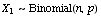be independent of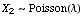. We seek the distribution of the sum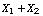. Solution: Let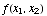denote the joint pmf of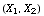: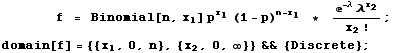Let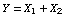and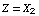. Then the joint pmf of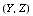, say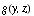, is given by mathStatica's Transform function as: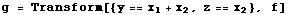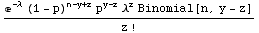Deriving the domain of support of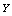and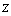is a bit more tricky. The following diagram plots the space in the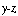plane where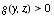.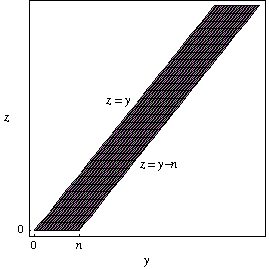Then the domain of support (the shaded region in the figure) can be defined as follows:     When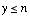: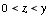When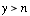: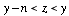The pmf ofis then obtained by summing outin each part of the domain: * If, the pmf of the sumis: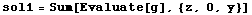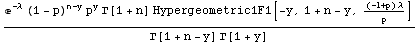* If, the pmf of the sumis: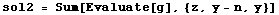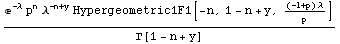where Hypergeometric1F1[a, b, z] denotes the Kummer confluent hypergeometric function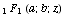.